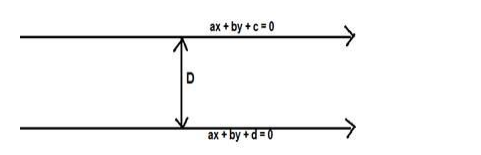# Find the distance between the parallel lines`
Question:

Find the distance between the parallel lines $y=m x+c$ and $y=m x+d$

Solution:

Given: parallel lines y = mx + c and y = mx + d

To find : distance between the parallel lines

Formula used: The distance between the parallel lines $a x+b y+c=0$ and $a x+b y+d$ $=0$ is,

$D=\frac{|d-c|}{\sqrt{a^{2}+b^{2}}}$The parallel lines are $m x-y+c=0$ and $m x-y+d=0$

Here $a=m, b=-1, c=c, d=d$

$D=\frac{|d-c|}{\sqrt{m^{2}+1^{2}}}=\frac{|d-c|}{\sqrt{m^{2}+1}}$

The distance between the parallel lines $y=m x+c$ and $y=m x+d$ is $\frac{|d-c|}{\sqrt{m^{2}+1}}$ units